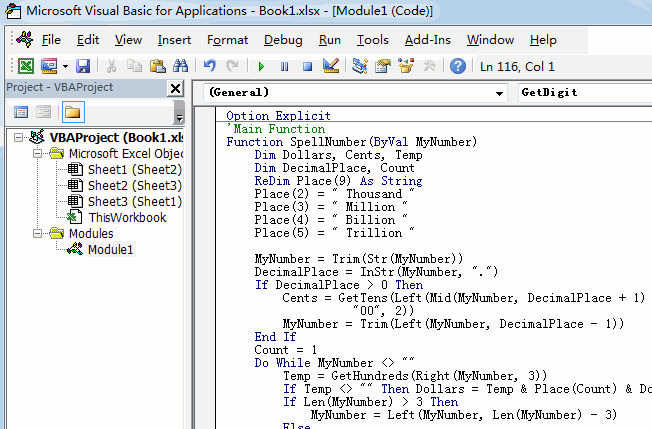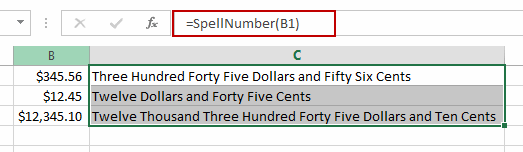# Convert Numbers into Words in Excel

This post will guide you how to convert numbers into words in Excel. How do I convert a numeric word into English words with VBA Function code in Excel. How to convert Numbers (Currency) to words with Excel VBA User Defined Function.

## Convert Numbers into Words

The Excel doesn’t have a default function that displays numbers as English words, but they saw that many users have this kinds of request, so they created and published a special VBA User Defined function called SpellNumber. So you can use this VBA Function to convert dollor and cent amounts to English words with a formula. Just do the following steps:

#1 open your excel workbook and then click on “Visual Basic” command under DEVELOPER Tab, or just press “ALT+F11” shortcut.#2 then the “Visual Basic Editor” window will appear.

#3 click “Insert” ->”Module” to create a new module.#4 paste the below VBA code into the code window. Then clicking “Save” button.```Option Explicit
'Main Function
Function SpellNumber(ByVal MyNumber)
Dim Dollars, Cents, Temp
Dim DecimalPlace, Count
ReDim Place(9) As String
Place(2) = " Thousand "
Place(3) = " Million "
Place(4) = " Billion "
Place(5) = " Trillion "

MyNumber = Trim(Str(MyNumber))
DecimalPlace = InStr(MyNumber, ".")
If DecimalPlace > 0 Then
Cents = GetTens(Left(Mid(MyNumber, DecimalPlace + 1) & _
"00", 2))
MyNumber = Trim(Left(MyNumber, DecimalPlace - 1))
End If
Count = 1
Do While MyNumber <> ""
Temp = GetHundreds(Right(MyNumber, 3))
If Temp <> "" Then Dollars = Temp & Place(Count) & Dollars
If Len(MyNumber) > 3 Then
MyNumber = Left(MyNumber, Len(MyNumber) - 3)
Else
MyNumber = ""
End If
Count = Count + 1
Loop
Select Case Dollars
Case ""
Dollars = "No Dollars"
Case "One"
Dollars = "One Dollar"
Case Else
Dollars = Dollars & " Dollars"
End Select
Select Case Cents
Case ""
Cents = " and No Cents"
Case "One"
Cents = " and One Cent"
Case Else
Cents = " and " & Cents & " Cents"
End Select
SpellNumber = Dollars & Cents
End Function

Function GetHundreds(ByVal MyNumber)
Dim Result As String
If Val(MyNumber) = 0 Then Exit Function
MyNumber = Right("000" & MyNumber, 3)
' Convert the hundreds place.
If Mid(MyNumber, 1, 1) <> "0" Then
Result = GetDigit(Mid(MyNumber, 1, 1)) & " Hundred "
End If
' Convert the tens and ones place.
If Mid(MyNumber, 2, 1) <> "0" Then
Result = Result & GetTens(Mid(MyNumber, 2))
Else
Result = Result & GetDigit(Mid(MyNumber, 3))
End If
GetHundreds = Result
End Function

Function GetTens(TensText)
Dim Result As String
Result = "" ' Null out the temporary function value.
If Val(Left(TensText, 1)) = 1 Then   ' If value between 10-19...
Select Case Val(TensText)
Case 10: Result = "Ten"
Case 11: Result = "Eleven"
Case 12: Result = "Twelve"
Case 13: Result = "Thirteen"
Case 14: Result = "Fourteen"
Case 15: Result = "Fifteen"
Case 16: Result = "Sixteen"
Case 17: Result = "Seventeen"
Case 18: Result = "Eighteen"
Case 19: Result = "Nineteen"
Case Else
End Select
Else ' If value between 20-99...
Select Case Val(Left(TensText, 1))
Case 2: Result = "Twenty "
Case 3: Result = "Thirty "
Case 4: Result = "Forty "
Case 5: Result = "Fifty "
Case 6: Result = "Sixty "
Case 7: Result = "Seventy "
Case 8: Result = "Eighty "
Case 9: Result = "Ninety "
Case Else
End Select
Result = Result & GetDigit _
(Right(TensText, 1))  ' Retrieve ones place.
End If
GetTens = Result
End Function

Function GetDigit(Digit)
Select Case Val(Digit)
Case 1: GetDigit = "One"
Case 2: GetDigit = "Two"
Case 3: GetDigit = "Three"
Case 4: GetDigit = "Four"
Case 5: GetDigit = "Five"
Case 6: GetDigit = "Six"
Case 7: GetDigit = "Seven"
Case 8: GetDigit = "Eight"
Case 9: GetDigit = "Nine"
Case Else: GetDigit = ""
End Select
End Function```

#5 Type the following formula into blank cells and then press Enter key.

=SpellNumber(B1)

Type this formula into a blank cell and then press Enter key in your keyboard. and then drag the AutoFill Handle over other cells to apply this formula.